2018-07-05 17:39:32 lmseo5hy 阅读数 416
• ###### Python数据分析实战-Pandas

深度学习、机器学习和数据分析必须用pandas。pandas是在python直接流行的数据处理框架。可以说，如果不会使用pandas，就谈不上会用python做数据分析。本课程会使用奥林匹克一个真实的数据作为实验数据，从初级到各种pandas的常用操作，到常用的数据可视化，让你在短的时间内掌握好pandas，轻松愉快的玩转数据分析。

2341 人正在学习 去看看 阿勒拉哈

```import numpy as np

x = np.arange(0,100)

y = x*2

z = x**2
```

1、导入matplotlib.pyplot 并命名为plt，如何支持Jupyter notebook内部绘图?非Jupyter otebook中如何绘图?

2、创建一个figure对象fig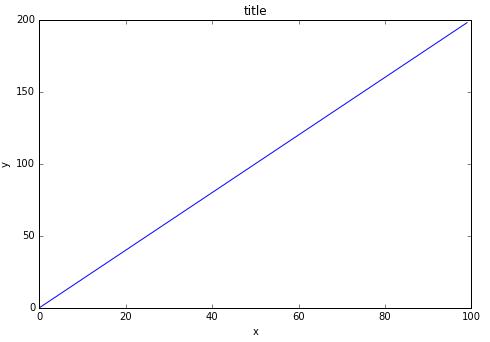4、创建一个figure对象，设置两个坐标轴ax1，ax2，位置分别为：[0,0,1,1] 和 [0.2,0.5,.2,.2]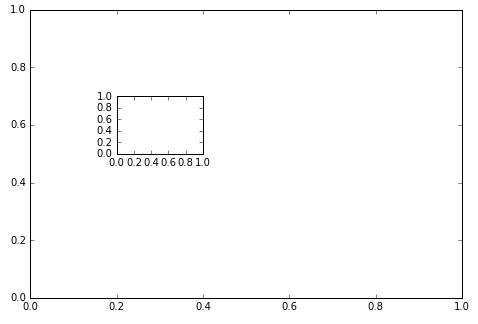5、调用plot (x,y) 绘制图形并显示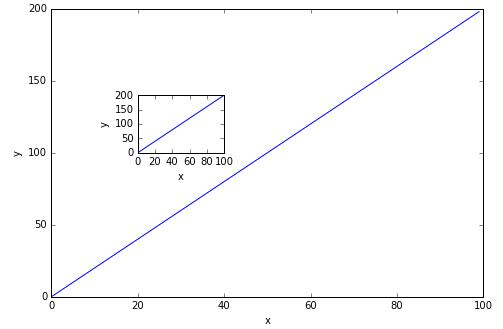6、创建figure对象，在 [0,0,1,1] 和 [0.2,0.5,.4,.4]创建两个坐标轴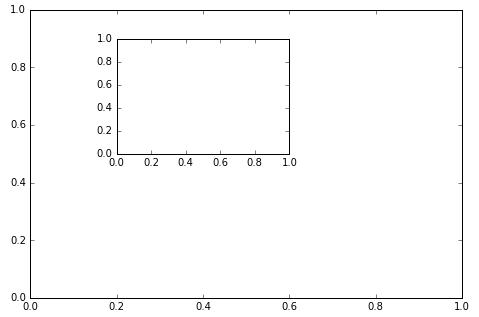7、使用x,y,z数组创建图形如下图所示，注意x，y的定义域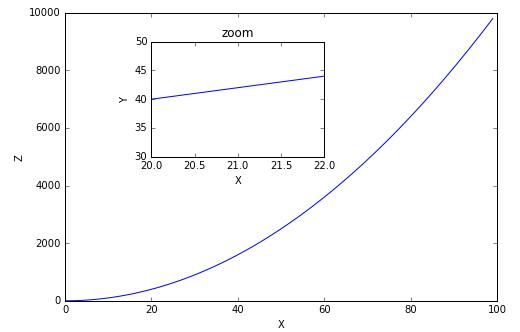8、使用plt.subplots(nrows=1, ncols=2) 创建如下图形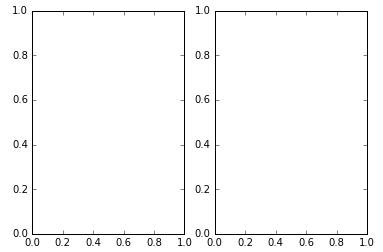9、使用plot (x,y) 和(x,z)并使用linewidth和style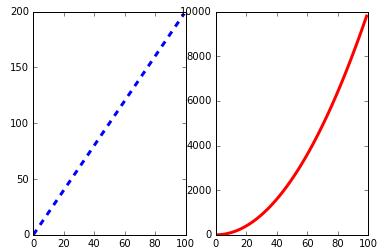10、重绘以上图形的大小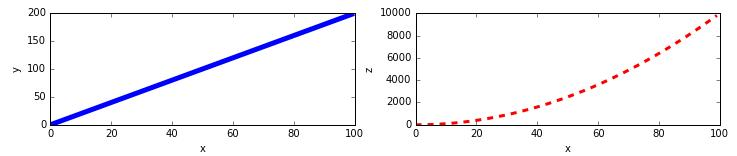python大数据分析练习题 相关内容

2019-05-27 16:14:10 qq_37635049 阅读数 3170
• ###### Python数据分析实战-Pandas

深度学习、机器学习和数据分析必须用pandas。pandas是在python直接流行的数据处理框架。可以说，如果不会使用pandas，就谈不上会用python做数据分析。本课程会使用奥林匹克一个真实的数据作为实验数据，从初级到各种pandas的常用操作，到常用的数据可视化，让你在短的时间内掌握好pandas，轻松愉快的玩转数据分析。

2341 人正在学习 去看看 阿勒拉哈

### 第一组 python基础语法：

（1）下面两段代码输出的结果相同么？请解释原因。
① x=4，y=5，print（x+y） ② x='4',y='5', print(x+y)

答：一是整数间加法运算,结果为 9 ；二是字符串之间的拼接操作，结果为 45。

（2）‘10/3'，‘10//3’和'10%3'结果相同么？请说明原因

答：结果分别为：3.333  ，3  ，1。其中，/是除法运算，//所得结果向下取整，也叫地板除，%是取余运算。

（3）string1='I'm a student.' 。print(string1)结果会报错么？如何修改string1使其得到正确的输出？

答：会报错SyntaxError: invalid syntax，因为系统会认为最近两个单引号是匹配的。如有特殊字符时候，可使用转义字符\ 。修改为 string1='I\'m a student.'     print(string1)

（4） a = 3，str(a*3) + str(a)*3的输出是什么?

答：输出结果为9333。str(a*3)得到的是字符串类型的 9，str(a)*3表示字符串 3 重复3次，加号是字符串拼接。

（5）my_string = 'this is my string', 如何查看这个字符串长度？

答：查看字符串长度用len(str)函数。即len(my_string)

（6）a,b = 4,5,a大于b 或 b大于等于5。请写出这里逻辑运算的代码，并查看输出会是什么。

答：a,b = 4,5     a>b or b>=5   ，输出结果为 True

（7）'='和'=='分别表示什么含义？print(2==5)输出什么结果？属于什么类型数据？

答：'='和'=='分别表示赋值和等于。print(2==5)输出False，属于布尔类型数据。

（8）计算：100%(2+2)**3//5

答：结果为7，计算步骤：100%4^3//5；100%64//5；36//5。其中**代表指数运算，%代表取余运算，//代表地板除（向下取整），同时注意计算次序。

（9）a,b = 4,5，a *= b，a=?

答：a=20，即a=4 ,b=5 ,a=a*b=4*5=20。

（10）int(3.14159)==float(3)会输出什么结果？为什么？type(int(3.14159))==type(float(3))的结果又是什么？

### 第二组 列表，字典，集合：

（1）L=[1,2,3,4,1,4,5,6],请完成：①删除列表中的重复元素并输出一个集合；②将7添加到集合中；③将集合转化为列表，删除元素3.

``````L=[1,2,3,4,1,4,5,6]
s=set(L)
print(s)``````

``````s.add(7)
print(s)``````

最后，列表用list()函数，删除元素用pop()函数。

``````L2 = list(s)
L2.pop(2)``````

（2）dog = ["tom", "jack", "Collie", "Marry"], kitty = "Marry", 如何判断 kitty 是否是 dog 中的一员?

答：使用in。in的常用用法：在 for 循环中，获取列表或者元组的每一项；判断左边的元素是否包含于列表（如本题中）；用来判断字符串是否包含某一串,可以用来筛选文件使用。返回结果为True 或 False。

``````dog = ["tom", "jack", "Collie", "Marry"]
kitty = "Marry"
kitty in dog``````

（3）dog = ["tom", "jack", "Collie", "Marry"]，将dog逆序。

``````(1)dog = ["tom", "jack", "Collie", "Marry"]
dog[ : : -1]

(2)dog = ["tom", "jack", "Collie", "Marry"]
dog.reverse()

(3)dog = ["tom", "jack", "Collie", "Marry"]
for i in reversed(dog):
print(i) ``````

（4）L=["former", "latter", "starboard"]，请完成：为L在末端添加一个元素“port”。

答：列表末端添加元素使用append()方法。

``````L1=["former", "latter", "starboard"]
L2 = ['nn','m',['nm']]

L1.append(L2)

L1.extend(L2)

L1.insert(0,L2)

L1+L2

（5）L=[1,2,3,1,5,11,3,6,4,2,5,8,4,2],计算L的最值，和加和。

答：最值L.max()，L.min()，加和L.sum()

（6）cube：length：40，width：40，height：40, 请创建一个字典，包括cube的所有信息，并向字典中添加条目“color”和对应值"red"，删除属性height，修改属性width为20.

答：创建字典的几种方式如。除此之外还可以通过推导式创建等。

``````#直接赋值创建，注意是大括号
dic = {'length':40,'width':40,'height':40}

#通过关键字dict和关键字参数创建
dic = dict（length=40, width=40, height=40）

#通过二元组列表创建
dic = [('length',40),('width',40),('height',40)]

#dict和zip结合创建
dic = dict(zip(['length','width','height'], [40, 40, 40]))``````

字典中增加元素：dict['color']='red'    ;

字典中删除元素：dict.pop('height')  ;

del dict['height'] # 删除键是'height'的条目

dict.clear() # 清空词典所有条目

del dict # 删除词典

字典中修改元素：dict['width']=20

（7）set_a = {1, 5, 10} set_b = {1, 10, 12} 找出set_a和set_b中共有的元素（交集）。

答：print(set_a&set_b)。交集 &或set_a.intersection(set_b)；并集| 或set_a.union(set_b)；差集- 或set_a.difference(set_b)。除此之外，还有对称差集和子集，超子集等。

（8）从1到10中选出所有的奇数。

答：可以使用切片方式实现。

``````import numpy as np
a = np.arange(1,11)
a[: : 2]``````

（9）将字典变为两个元素一一对应的列表，一个只含有关键字，另一个只含有值。 如:{'a': 1, 'b': 2}变为['a', 'b'], [1, 2]

答：使用keys()和values()函数实现。

``````dt = {'a': 1, 'b': 2}
a = dt.keys()
b = dt.values()``````

## 第三组 条件判断，循环和函数

（1）L=[1,2,'','my',3,'name','is',4,'katty'],利用循环语句和判断条件，分别输出列表中的字符串和数字。

答：循环语句for循环和whlie循环；判断语句if...else。

``````L=[1,2,'','my',3,'name','is',4,'katty']
for i in L:
if type(i)==int:
print(i)
else:
continue

for i in L:
if type(i)==str:
print(i)
else:
continue``````

（2）利用循环语句输出1到50中5的倍数，将其存放到一个列表中。

``````a=[]
for i in range(1,51):
if i%5==0:
a.append(i)
print(a)
``````

（3）定义一个判断字符串的长度是否大于10的函数。

答：定义函数

• 函数代码块以 def 关键词开头，后接函数标识符名称和圆括号()
• 任何传入参数和自变量必须放在圆括号中间。圆括号之间可以用于定义参数。
• 函数的第一行语句可以选择性地使用文档字符串—用于存放函数说明。
• 函数内容以冒号起始，并且缩进。

return [表达式] 结束函数，选择性地返回一个值给调用方。不带表达式的return相当于返回 None。

``````def fun(s):
if len(s)>10:
print('字符串长度大于10')
else:
print('字符穿串长度不大于10')
s='hello world,i love you'
fun(s)``````

（4）定义一个求解阶乘的函数。

``````import numpy as np
import pandas as pd
from functools import reduce
def factorial(n):
L = np.arange(1,n+1)
return reduce(lambda x,y:x*y,L)
factorial(5)``````

答：reduce()函数是内置函数，使用的方式：reduce(function, iterable[, initializer])，其中function表示函数，有两个参数；iterable 表示可迭代对象；initializer表示可选，初始参数。

（5）利用列表生成式，生成1-5的阶乘。

``[factorial(i) for i in range(1,6)]``

答：使用到了（4）中的函数。

（6）利用函数和列表生成式，标记一个列表，奇数标记为1，偶数标记为2，并且统计一下奇数和偶数的数量。 例如：[1,4,2,4,2,9,5]，得到[1,2,2,2,2,1,1]

``````函数：
def func(i):
if i%2==0:
return 2
else:
return 1
l = [1,4,2,4,9,5]

[func(i) for i in l]``````

python大数据分析练习题 相关内容

2020-01-20 21:36:03 hongwangdb 阅读数 37
• ###### Python数据分析实战-Pandas

深度学习、机器学习和数据分析必须用pandas。pandas是在python直接流行的数据处理框架。可以说，如果不会使用pandas，就谈不上会用python做数据分析。本课程会使用奥林匹克一个真实的数据作为实验数据，从初级到各种pandas的常用操作，到常用的数据可视化，让你在短的时间内掌握好pandas，轻松愉快的玩转数据分析。

2341 人正在学习 去看看 阿勒拉哈

### Python练习题（四）

1. 题目：股票市场分析
输出1：阿里及亚马逊两者走势图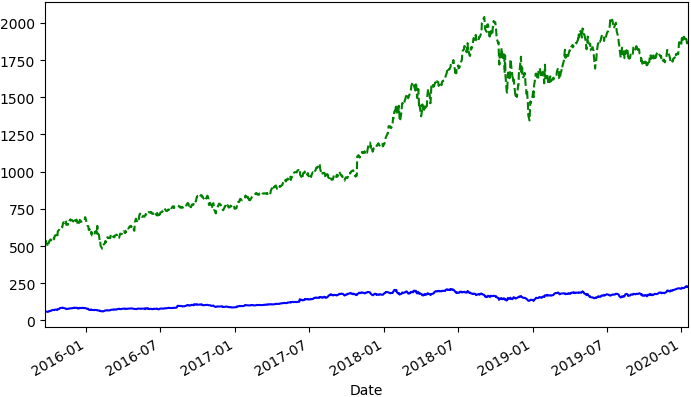输出2：阿里每日变化情况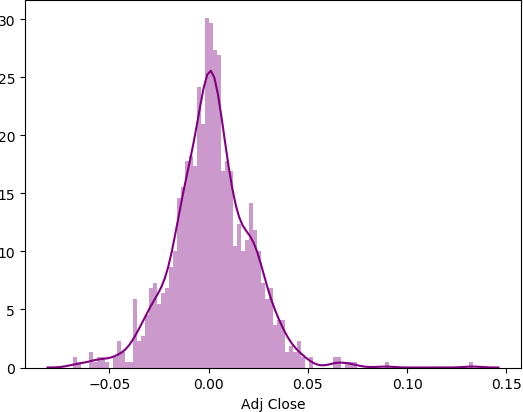输出3：五家公司的价格走势图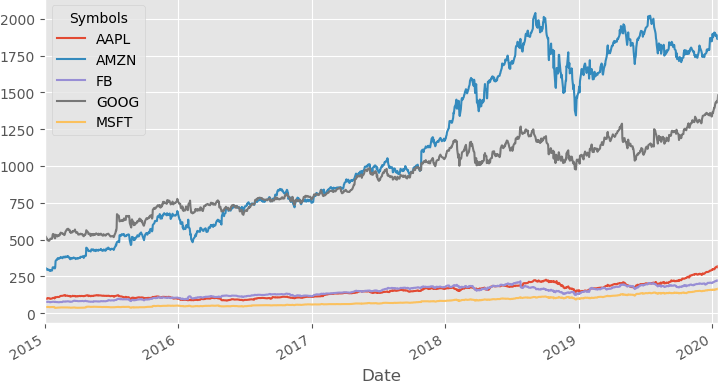输出4：亚马逊及谷歌 展示pearsonr，p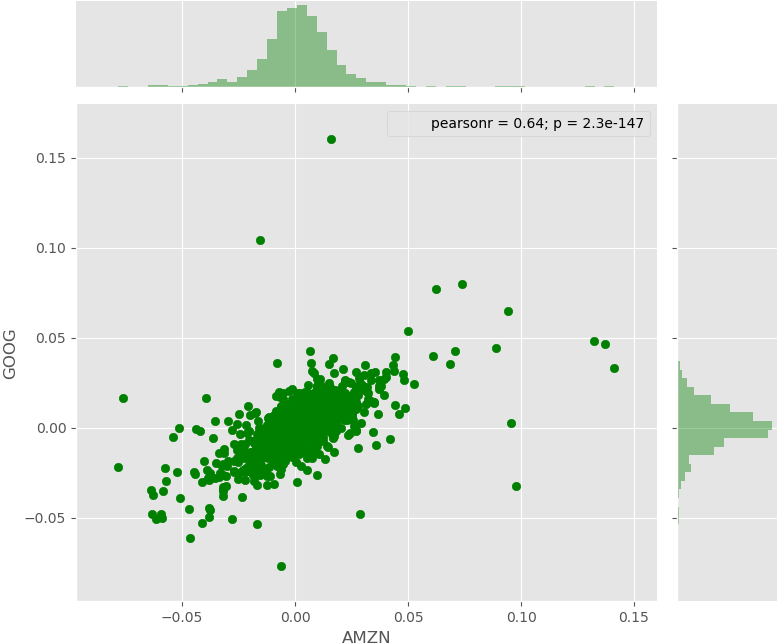输出5：亚马逊及谷歌 展示R^2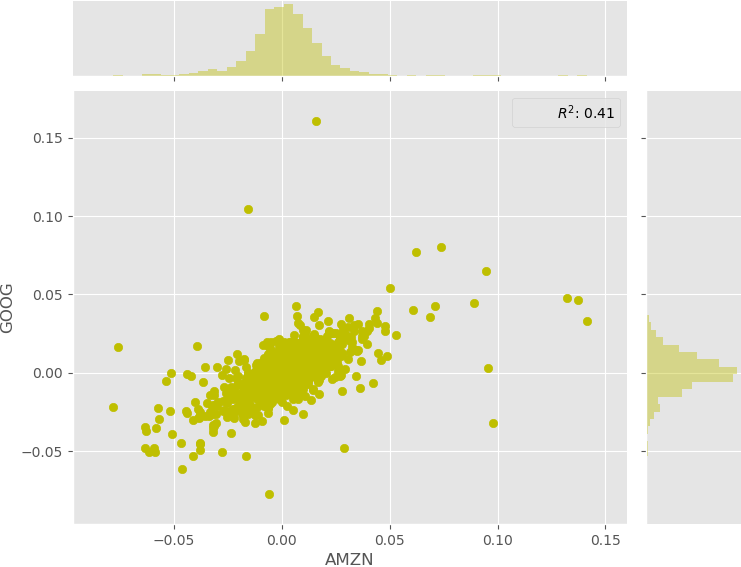输出6：多个子图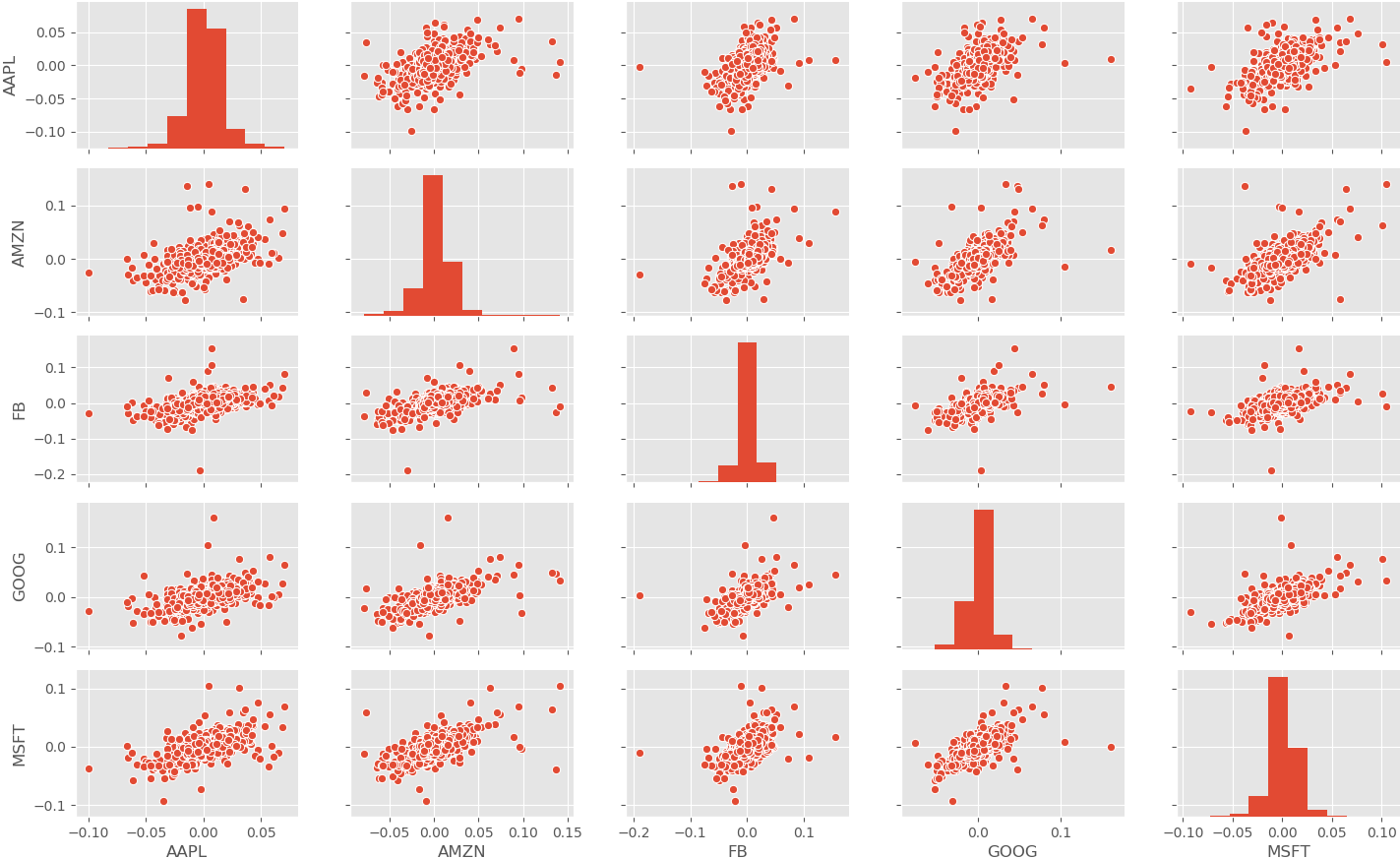输出7：

有48%的信心对于AAPL最大亏损是0.0013426490233132958
有95%的信心对于MSFT最大亏损是-0.020935521929566915
请按任意键继续. . .

代码：

``````		import numpy as np
import pandas as pd
from pandas import Series, DataFrame
# 股票数据获取
# 可视化
import matplotlib.pyplot as plt
import seaborn as sb
# time
from datetime import datetime
# Add an annotation with a statistic summarizing the bivariate relationship
from scipy import stats

# ----------------------------------------------------
'''start = datetime(2015, 9, 20)
alibaba = pdr.get_data_yahoo('BABA', start=start)
amazon = pdr.get_data_yahoo('AMZN', start=start)

# 1.两者走势图
# 2.alibaba每天变化情况

plt.show()'''
# ----------------------------------------------------
start = datetime(2015, 1, 1)
company = ['AAPL', 'GOOG', 'MSFT', 'AMZN', 'FB']  # 苹果/谷歌/微软/亚马逊/脸书
top_tech_df_b = top_tech_df_a.pct_change()

plt.style.use('ggplot')

# 3.五家公司的价格走势图,若只需要其中三家,代码可top_tech_df_a['AAPL', 'FB', 'MSFT'].plot()
# top_tech_df_a.plot()

# 4.展示 pearsonr,p
# sb.jointplot('AMZN', 'GOOG', top_tech_df_b, kind='scatter', color='g').annotate(stats.pearsonr)
# 5.展示 R^2
# rsquare = lambda a, b: stats.pearsonr(a, b) ** 2
# sb.jointplot('AMZN', 'GOOG', top_tech_df_b, kind='scatter', color='y').annotate(rsquare, template="{stat}: {val:.2f}", stat="\$R^2\$")
# 6.多个子图
sb.pairplot(top_tech_df_b.dropna())

plt.show()

# 7.风险评估
a = top_tech_df_b['AAPL'].quantile(0.52)
b = top_tech_df_b['MSFT'].quantile(0.05)
print('有48%的信心对于AAPL最大亏损是{}\n有95%的信心对于MSFT最大亏损是{}'.format(a, b))
``````

### 未完待续，，，

python大数据分析练习题 相关内容

2019-05-27 17:44:29 qq_37635049 阅读数 621
• ###### Python数据分析实战-Pandas

深度学习、机器学习和数据分析必须用pandas。pandas是在python直接流行的数据处理框架。可以说，如果不会使用pandas，就谈不上会用python做数据分析。本课程会使用奥林匹克一个真实的数据作为实验数据，从初级到各种pandas的常用操作，到常用的数据可视化，让你在短的时间内掌握好pandas，轻松愉快的玩转数据分析。

2341 人正在学习 去看看 阿勒拉哈

说明：以练习题的方式，学习python基础知识点，主要是数据分析方向常用的numpy、pandas、matplotlib等库对应的练习题。仅供参考，题目来源于网络，如有侵权请联系我删除。

### numpy数组部分：

（1）创建一个1到10的数组，然后逆序输出。

答：实现数组逆序方法包括切片，reversed()函数和自定义函数实现。对比习题（一）中列表逆序输出，想想区别。

``````#切片
import numpy as np
a=np.arange(1,11)
a[: : -1]``````
``````#reversed（）函数
import numpy as np
a=np.arange(1,11)
for i in a.reversed():
print(i)``````

（2）创建一个长度为20的全1数组，然后变成一个4×5的二维矩阵并转置。

答：创建全1数组采用ones()函数，改变矩阵形状用reshape()函数。查看矩阵形状用shape()函数。可试试区分以下函数：shape(),reshape(),type(),dtype()

【shape()查看形状，reshape()改变形状，type()数组类型，dtype()数组中元素类型】

``````import numpy as np
#生成全1数组
b = np.ones(20,dtype=int)
#改变数组形状
b.reshape(4,5)``````

（3）创建一个3x3x3的随机数组。 (提示: np.random.random)

答：如代码所示。注意括号，尝试区分random(),randn(),randint()。

【random()返回随机生成的一个实数，它在[0,1)范围内；randint()随机生一个整数int类型，可以指定这个整数的范围，同样有上限和下限值；randn()以给定的形状创建一个数组，数组元素来符合标准正态分布N(0,1) 】

``````import numpy as np
c = np.random.random((3,3,3))``````

（4）从1到10中随机选取10个数，构成一个长度为10的数组，并将其排序。获取其最大值最小值，求和，求方差。

答：排序sort()函数，最大值max()函数，最小值min()，求和sum()函数，方差var()函数。除此之外还可以采用切片的方式从排序后的数组中取出最大值最小值（即：a[-1]和a）

``````import numpy as np
#生成数组
a = np.random.randint(1,11,10)
#排序
a.sort()
#最大值
a.max()
#最小值
a.min()
#方差
a.var()``````

（5）从1到10中随机选取10个数，构成一个长度为10的数组，选出其中的奇数。

答：如代码所示。其中b%2==1返回值是布尔类型，即True 或 False

``````import numpy as np
b = np.random.randint(1,11,10)
b[b%2==1]``````

（6）生成0到100，差为5的一个等差数列，然后将数据类型转化为整数。

答：生成等差数列采用linspace()函数，转换数据类型采用astype()函数。

``````import numpy as np
#生成等差数据
a = np.linspace(0,100,21)
#改变类型
a.astype('int')``````

（7）从1到10中随机选取10个数，大于3和小于8的取负数。（可参考(5)题答方法想想）

``````import numpy as np
a = np.random.randint(1,11,10)
a[(a>3)&(a<8)]*=-1``````

（8）在数组[1, 2, 3, 4, 5]中相邻两个数字中间插入1个0。

答：如果直接插入0，则会涉及数组中元素的后移操作等。所以换一种思路。插入0后形成共9个元素的数组，先生成一个全零长度为9的数组。

``````import numpy as np
a=[1,2,3,4,5]
b =np.zeros(9,dtype='int')
b[: : 2]=a``````

（9）新建一个5乘5的随机二位数组，交换其中两行？比如交换第一二行。

答：注意randint()中第三个参数，注意交换行操作a[[1,0,2,3,4]]。

``````import numpy as np
#生成二维数组
a = np.random.randint(1,10,(5,5))
#交换第一行和第二行
a=a[[1,0,2,3,4]]
``````

（10）把一个10*2的随机生成的笛卡尔坐标转换成极坐标。

答：区别笛卡尔坐标与极坐标概念。笛卡尔坐标就是（x,y）;极坐标是（r,R）【百度下极坐标吧】

``````import numpy as np
#笛卡尔坐标
a = np.random.randint(1,10,(10,2))
#极坐标
x = a[:,0]
y = a[:,1]
r = np.sqrt(x**2+y**2)  #距原点距离
R = np.arctan(y/x)  #弧度``````

（11）创建一个长度为10并且除了第五个值为1其余的值为2的向量。(全1向量乘以2就是啦)

``````import numpy as np
a = np.ones(10,dtype='int')*2
a=1``````

（12）创建一个长度为10的随机向量，并求其累计和。

答：注意累加和哦，使用cumsum()函数。

``````import numpy as np
a = np.random.randint(1,11,10)
a.cumsum()``````

（13）将数组中的所有奇数替换成-1。(对比题(5)，再想想)

``````import numpy as np
a=np.random.randint(1,11,10)
a[a%2==1] = -1``````

（14）构造两个4乘3的二维数组，按照3种方法进行连接？

答：二维数组连接，vstack()函数，hstack()函数，dstack()函数。也可以使用stack()函数，配合axis=0，1参数使用，完成数组连接，例如np.stack((a,b),axis=0)。

``````import numpy as np
a = np.random.randint(1,10,(4,3))
b = np.random.randint(1,10,(4,3))
np.vstack((a,b))
np.hstack((a,b))
np.dstack((a,b))``````

（15）获取数组 a 和 b 中的共同项（索引位置相同，值也相同）。 a = np.array([1,2,3,2,3,4,3,4,5,6])，b = np.array([7,2,10,2,7,4,9,4,9,8])

答：因为需要索引相同，值相同。考虑数组与数组间相减运算。

``````import numpy as np
a = np.array([1,2,3,2,3,4,3,4,5,6])
b = np.array([7,2,10,2,7,4,9,4,9,8])
c = a-b
a[c==0]``````

（16）从数组 a 中提取 5 和 10 之间的所有项。a=np.array([7,2,10,2,7,4,9,4,9,8])

答：我也不确定题目中 5 和 10 之间是指索引还是数值，所以分别如下：

``````import numpy as np
a=np.array([7,2,10,2,7,4,9,4,9,8])
#索引
a[5:10]

#数值
a[(a>=5)&(a<=10)]``````

注：数组部分结束。如果想自己练习，可以到我的百度网盘下载原题，使用Jupyter notebook自己写python代码实现。加油哦！

python大数据分析练习题 相关内容

2019-05-28 17:20:15 qq_37635049 阅读数 290
• ###### Python数据分析实战-Pandas

深度学习、机器学习和数据分析必须用pandas。pandas是在python直接流行的数据处理框架。可以说，如果不会使用pandas，就谈不上会用python做数据分析。本课程会使用奥林匹克一个真实的数据作为实验数据，从初级到各种pandas的常用操作，到常用的数据可视化，让你在短的时间内掌握好pandas，轻松愉快的玩转数据分析。

2341 人正在学习 去看看 阿勒拉哈

说明：以练习题的方式，学习python基础知识点，主要是数据分析方向常用的numpy、pandas、matplotlib等库对应的练习题。仅供参考，题目来源于网络，如有侵权请联系我删除。

### Pandas中的统计分析与透视表：

（1）读取上次作业保存的数据，酒店数据1.xlsx

``````import numpy as np
import pandas as pd

（2）查看“评分”的格式，并分别进行升序和降序排序

答：查看格式,查看元素类型均为.dtype。排序函数sort_values()，升序ascending=True，降序ascending=False。

``````#查看 评分 的格式
data['评分'].dtype

#升序，默认升序
data.sort_values('评分')

#降序
data.sort_values('评分',ascending=False) ``````

（3）对酒店按照价格进行排名，计算“油尖旺”地区的均价。

答：排序sort_values()，计算均值mean()

``````#按价格排序
data.sort_values('价格')
#计算某地区均价
data[data['地区']=='油尖旺'].价格.mean()``````

（4）对酒店数据进行描述性统计，并求所有价格的均值方差，最大最小值，中值。

答：描述性统计函数describe()。

``data['价格'].describe()``

（5）计算评分和价格之间的的相关系数，协方差

答：相关系数corr()，协方差cov()。

``````#相关系数计算
data['评分'].corr(data['价格'])

#协方差计算
data['评分'].cov(data['价格'])``````

（6）按照评分降序排序，评分相同时按价格升序排序。

答：多个属性排序，且排序方式不同。

``data.sort_values(['评分','价格'],ascending=(False,True))``

（7）计算一下，评分小于3分的酒店数量和占比。

答：统计数量可以调用count()函数，或者用len()函数。

``````#评分小于3的数据量
len(data[data['评分']<3])
#占比
len(data[data['评分']<3])/len(data)``````

（8）计算一下，酒店评分大于等于4分的酒店的价格均值。

``data[data['评分']>=4].价格.mean()``

（9）计算出每个地区的酒店占总酒店数量的比例。

答：value_counts()方法是Series类型的方法，DataFrame类型没有。但是DataFrame类型有count()方法。

``````#每个地区酒店的数量
data['地区'].value_counts()

#占比
data['地区'].value_counts()/len(data)``````

（10）找出酒店评分人数排名前20的酒店，并计算他们的价格均值。

答：使用切片实现排名前20，计算均值用mean()。

``data.sort_values('评分人数',ascending=False)[:20]['价格'].mean()``

（11）查看酒店分布的类型数量和地区数量，并统计各个类型和地区包含的酒店数量。

``````#查看类型
data['类型'].unique()
#类型的数量
len(data['类型'].unique())

#查看地区
data['地区'].unique()
#查看地区数量
len(data['地区'].unique())

#统计各个类型和地区包含的酒店数量
data['类型'].value_counts()
data['地区'].value_counts()
``````

（12）用数据透视表，计算每个类型的酒店的评分人数总数量。

答：数据透视表pd.pivot_table(参数1[数据]，参数2[index索引]，参数3[values值]，其他参数方法等)

``pd.pivot_table(data,index=['类型'],values=['评分人数'],aggfunc=np.sum)``

（13）用数据透视表，计算每个类型的酒店价格的均值和标准差

``pd.pivot_table(data,index=['类型'],values=['价格'],aggfunc=[np.mean,np.std])``

（14）用数据透视表，计算每个地区酒店价格和评分的最大值和最小值

``pd.pivot_table(data,index=['类型'],values=['价格','评分'],aggfunc=[np.max,np.min])``

（15）用数据透视表，计算每个地区和类型的酒店的评分的均值和标准差

``pd.pivot_table(data,index=['地区','类型'],values=['评分'],aggfunc=[np.mean,np.std])``

注：第四部分结束。如果想自己练习，可以到我的百度网盘下载原题，使用Jupyter notebook自己写python代码实现。加油哦！

python大数据分析练习题 相关内容

阅读数 397

博文 来自： qq_37635049

阅读数 1695

博文 来自： qq_41794348

阅读数 624

博文 来自： qq_37635049

阅读数 333

博文 来自： qq_37635049

阅读数 3632

博文 来自： EGEFCXzo3Ha1x4
没有更多推荐了，返回首页